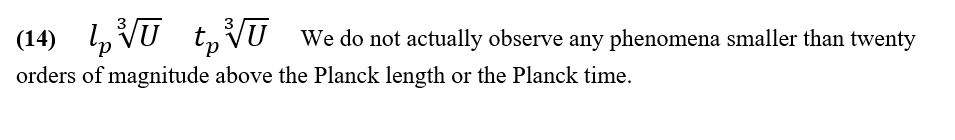## Hypersphere CosmologyAlternative to big-bang

Monday, 22 February 2021 16:57

## Equation 14Equation 14

The universe has an information deficit in the sense that it has only one bit of information/entropy for every e20 Planck lengths and this represents the universe’s pixilation or ‘grain size’. In practise we do not observe anything physically real at LESS than twenty orders of magnitude Above the Planck Length or Above the Planck Time.

See below: -

More in this category: « Equation 15 Equation 13 »
• #### HyperSphere Cosmology +Hypersphere Cosmology. (6)    P J Carroll 28/2/2021   Abstract.
• #### Type 1A supernovae and Hypersphere Cosmology +

Lensing, Redshift and Distance for Type1A Supernovae. All data input from Perlmutter et all, https://arxiv.org/pdf/astro-ph/9812133.pdf The measured Apparent Magnitudes of
• #### Galactic Rotation Curves +

Galactic Rotation Curves. A large discrepancy exists between the rotation curves for disc galaxies expected from a classical or relativistic
• #### Equation 17 +

Equation 17 The Redshift-Distance and the Lensing equations 6 and 16 together yield a derivation for the Antipode length L
• #### Equation 16 +

Equation 16 A stereographic projection can show the effect of projecting an n-sphere into an n-dimensional space. For
• #### Equation 15 +

Equation 15 It may seem odd that whilst the ‘enhanced’ Planck length and Planck time set a lower limit for
• #### Equation 14 +

Equation 14 The universe has an information deficit in the sense that it has only one bit of information/entropy for
• #### Equation 13 +

Equation 13 The universe has an information deficit in the sense that it has only one bit of information/entropy for
• #### Equation 12 +

Equation 12 The Bekenstein-Hawking conjecture arose to explain the behaviour of entropy in a black hole. https://en.wikipedia.org/wiki/Black_hole_thermodynamics#Overview It asserts that
• #### Equation 11 +

Equation 11 The Dirac Large Numbers Hypothesis suggests that a huge dimensionless number, or perhaps just a few huge dimensionless
• #### Equation 10 +

Equation 10 Here we show the modification to Newtonian Dynamics expected in a positively curved spacetime that has a negative
• #### Equation 9 +

Equation 9 Galaxies rotate but they do not rotate in accordance with our standard ideas about gravity. A large discrepancy
• 1
• 2